##### How to Use MAX() Function in PostgreSQL

PostgreSQL offers a built-in MAX() function that is used to retrieve the maximum value of a set. The MAX() function has multiple real-life implementations, such as fetching the highest-paid employee, finding a top-ranked student, and so on. So, let’s learn the working of the MAX() function through Practical examples.

How to Use MAX() Function in Postgres?

The below snippet will show you the basic syntax of the MAX() function:

`MAX(exp);`

Here, exp represents an expression. Let’s practically implement the MAX() function to get profound knowledge about it.

Example #1: How to Use MAX() Function on Table’s Data?

Suppose we have a table named bike_details. Let’s run the SELECT statement to get all the records of the bike_details table:

`SELECT * FROM bike_details;`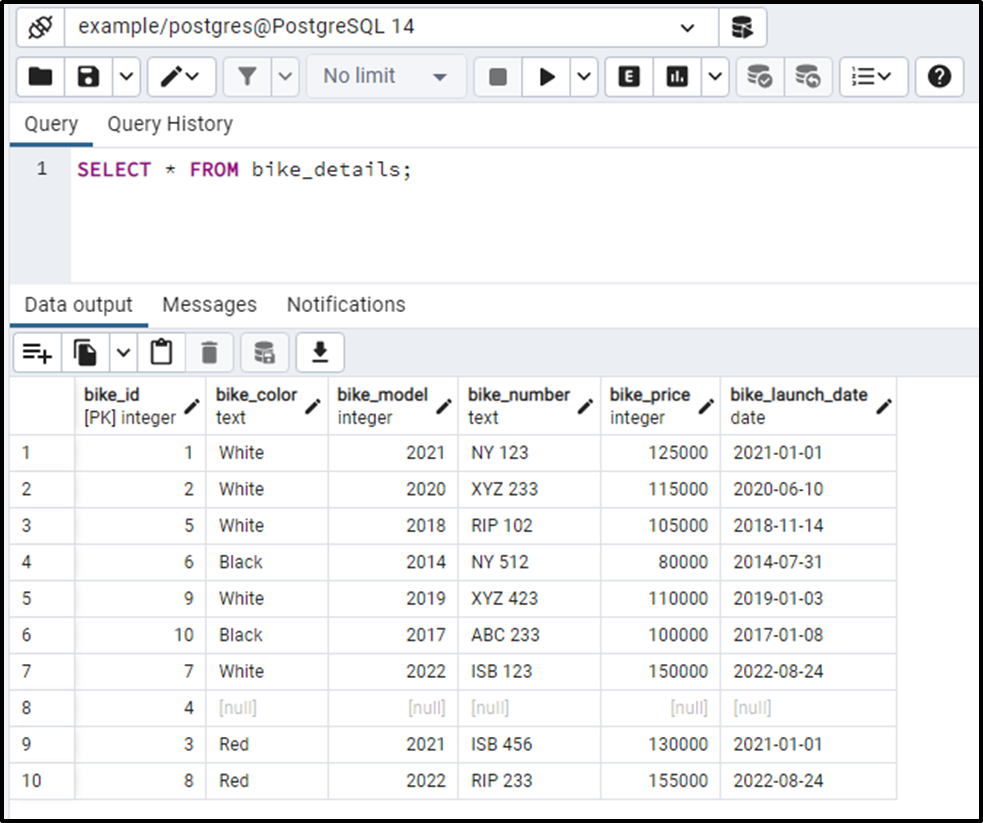Let’s find the bike that has the maximum price using the MAX() Function:

```SELECT MAX(bike_price)
FROM bike_details;```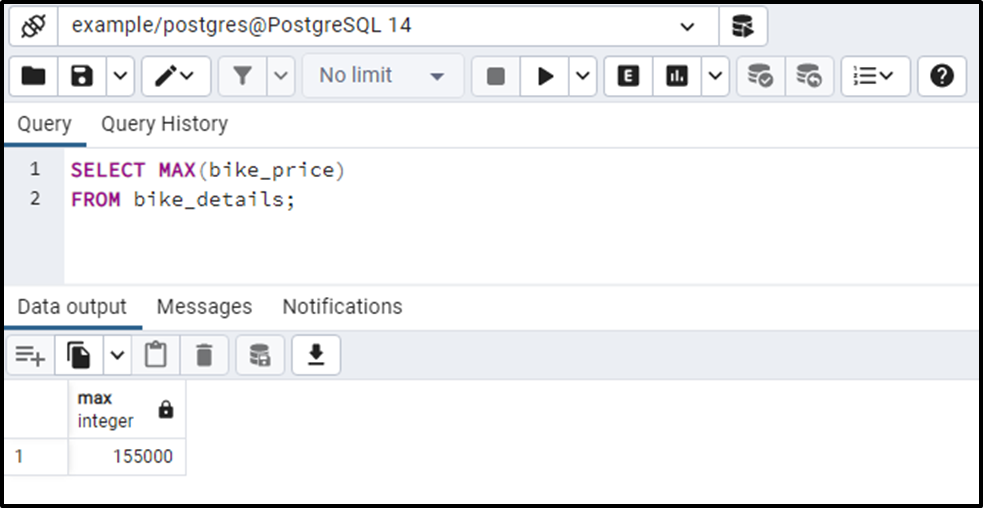The output shows that in the bike_details table, the most expensive bike costs 150000.

Example #2: How to Use MAX() Function With a Subquery?

Suppose we want to fetch all the details about the most expensive bike. To do so, we can use the MAX() function with the subquery as follows:

```SELECT * FROM bike_details
WHERE bike_price = (
SELECT MAX (bike_price)
FROM bike_details
);```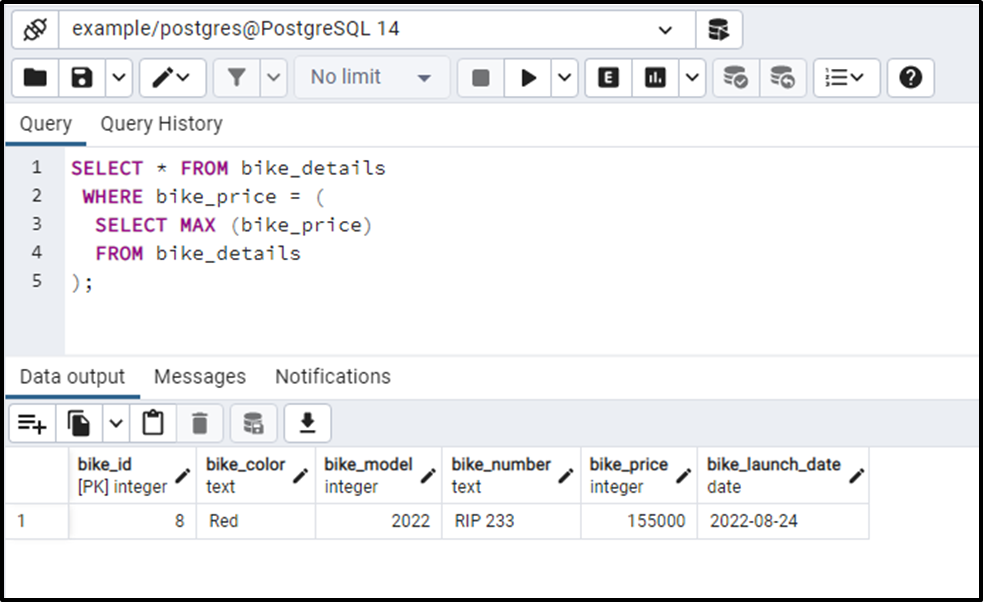This time, we got detailed information about the desired bike(bike having the highest price).

Example #3: How to Use the MAX() Function With GROUP BY Clause?

The following command will return the bike with the highest price from each group. Let’s utilize the GROUP BY clause as follows:

```SELECT
bike_model,
MAX (bike_price)
FROM bike_details
GROUP BY bike_model;```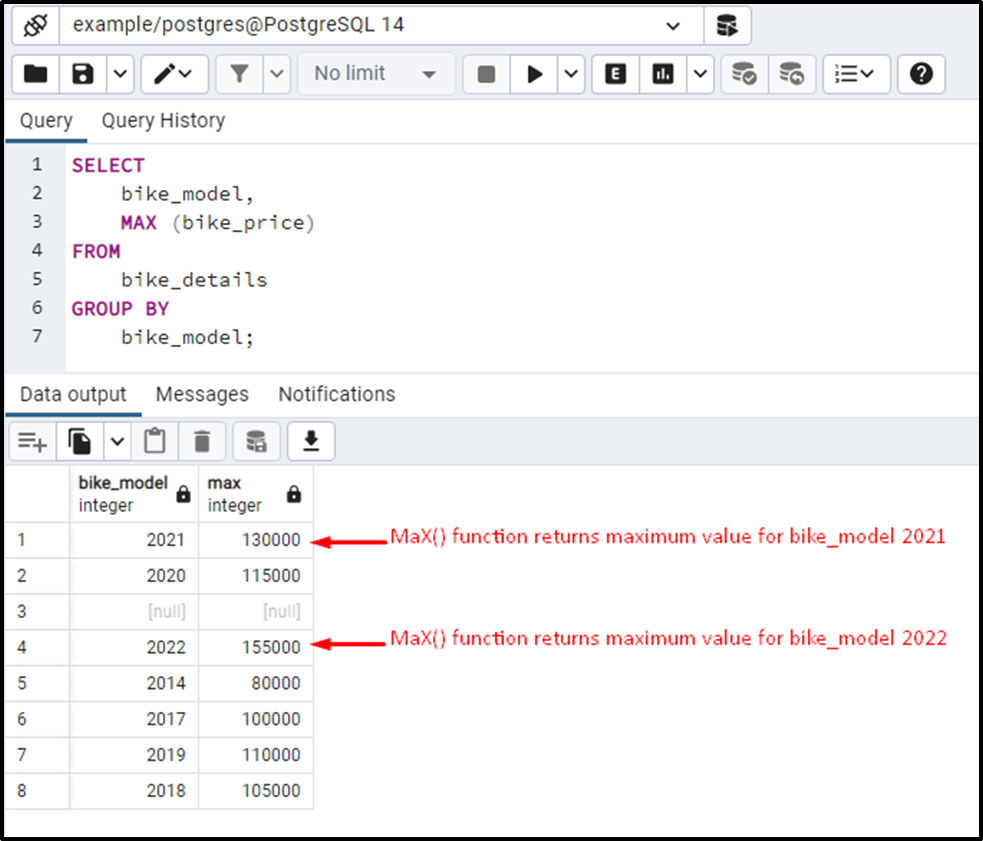From the output, you can observe that this time the MAX() function returned the maximum value for each group.

Example #4: How to Use the MAX() Function With HAVING Clause?

We can specify a special condition with the MAX() function using the HAVING clause:

```SELECT
bike_model,
MAX (bike_price)
FROM
bike_details
GROUP BY
bike_model
HAVING MAX(bike_price) >100000;```

In this example, we utilized the MAX() function along with the GROUP BY clause to find the bike from each group that has the highest price. Next, we utilized the HAVING clause specifying that the bike price must be greater than 100000. Here is the final outcome of the above query: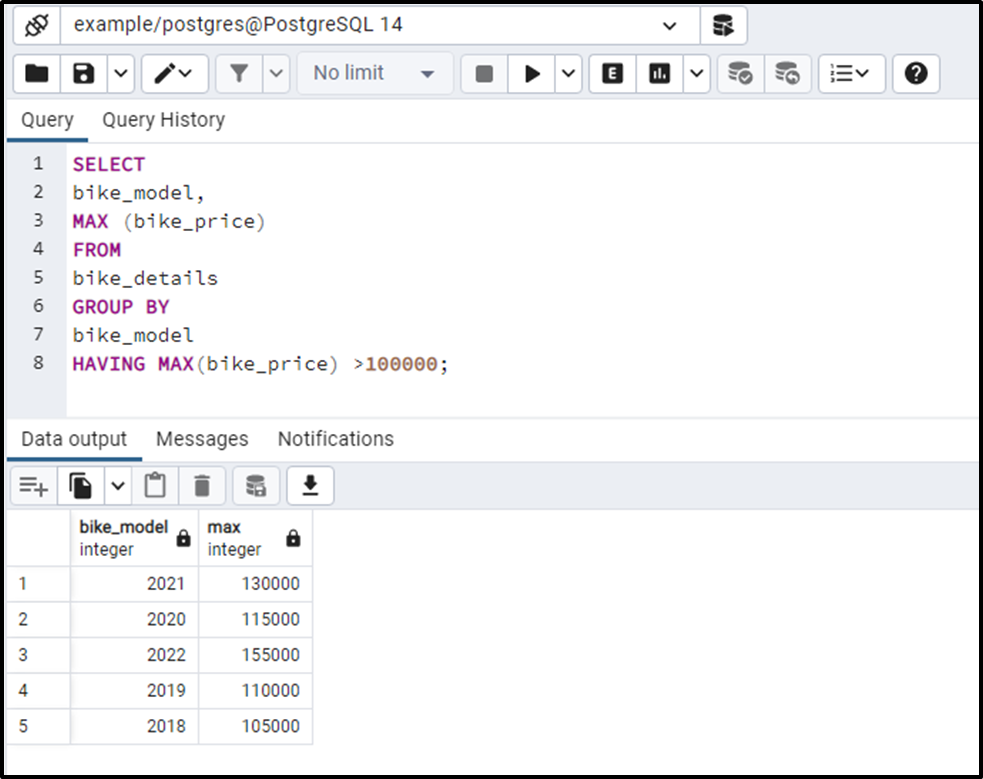The output shows that the MAX() function retrieves the bikes having the highest price in their respective group. However, it skipped the bikes having a price less than or equal to 100000.

Example #5: How to Get the Maximum Value From Multiple Columns?

Use the GREATEST() function to get the maximum/greatest value from more than one column. We have modified the bike_details table by inserting a new column in it. The below-given command will show the modified table:

`SELECT * FROM bike_details;`

Now, we will utilize the GREATEST() function to fetch the greatest value from the bike_price and bike_new_price columns: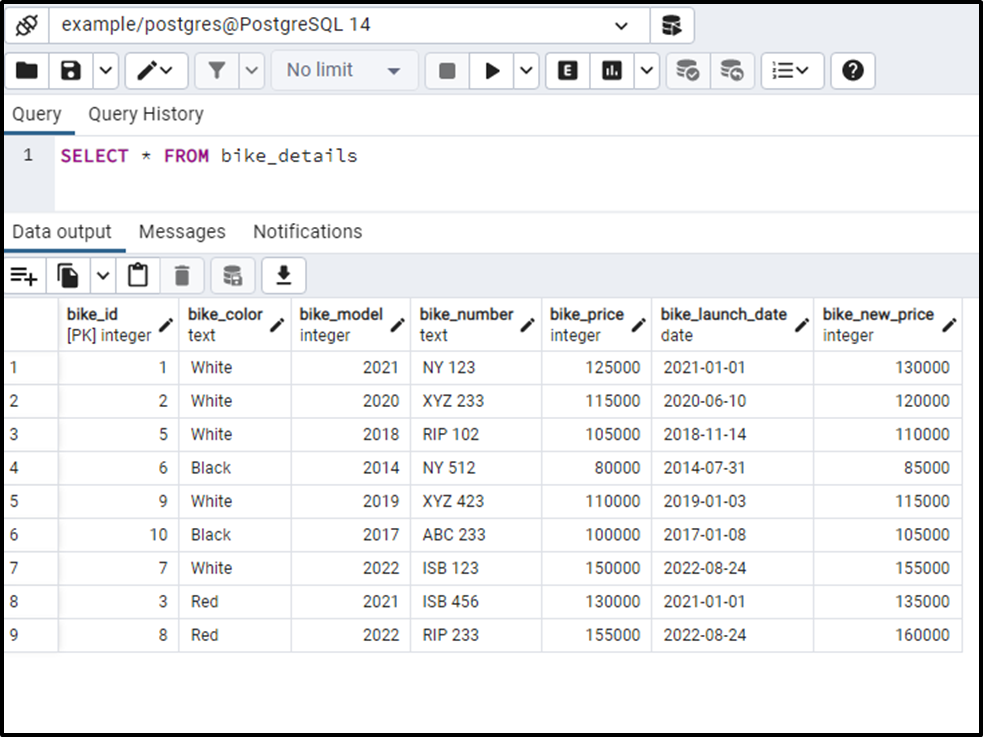```SELECT
bike_model,
GREATEST (bike_price, bike_new_price) AS greatest_price
FROM
bike_details;```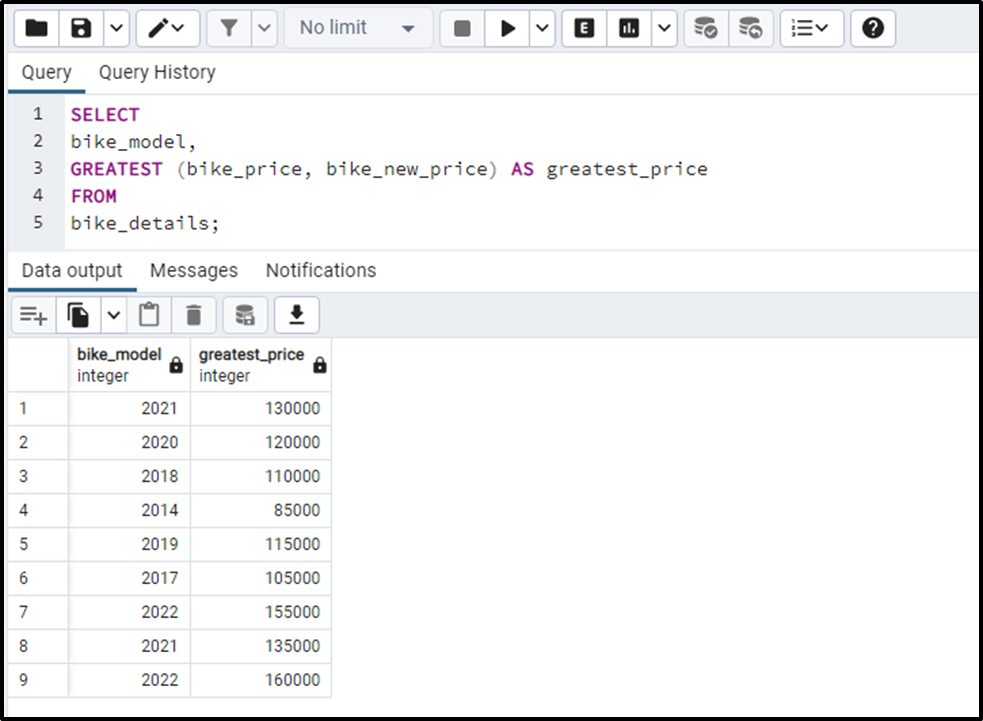This is how the GREATEST() function works in PostgreSQL.

Conclusion

PostgreSQL provides an in-built function named MAX() that is used to retrieve the maximum value of a set. The MAX() function has various use cases, such as fetching the highest-paid employee, finding a top-ranked student, etc. In this write-up, we have learned how to use the MAX() function with the HAVING clause, GROUP BY clause, and subquery. This write-up considered different examples to demonstrate the working of the MAX() function in PostgreSQL.